# Set-Builder Notation

Recall that, by definition,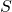being a set is equivalent to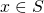" being an open sentence. This means that the theory of sets and the theory of logic are basically the same--differing only by notation and perspective. One can exploit this correspondence by using open sentences to define sets. Given an open sentence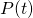, we can define the corresponding set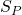, which consists of all valuesso thatis true. We write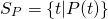and read aloudis the set of allsuch that." In other words,is the set so that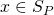means exactly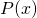. This way of defining sets has the unfortunately puerile name set-builder notation. It's how most sets in most mathematics texts are defined. By way of example, we'll rephrase some previous definitions.

Definition.

•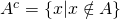•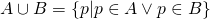Activity Complete the list of set operations by giving set-builder descriptions of each of the following set operations.

•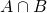•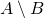•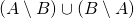•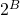Exercise. Consider two sets,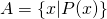and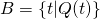. What can we say about the open sentences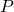and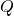if we know each of the following facts about the sets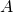and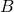?

•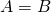•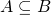•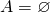•is the universe

The last exercise referenced the universe, which naturally leads to the question of how to specify the universe in set-builder notation. An even number is an integer which is twice some other integer. So we could write

Definition.

*** QuickLaTeX cannot compile formula:
\begin{equation*}\left\{n\middle\vert n\in\mathbb{Z}\wedge\exists k:\left(k\in \mathbb{Z}\wedge n=2k\right) \right\}
But the right-hand side (after the pipe symbol $\vert$) is a little crowded. Doing the same trick as we did in, we want to handle the conditions $n\in\mathbb{Z}$ and $k\in\mathbb{Z}$ by changing the universe. We do this as follows:
<strong>Definition. </strong>The set of even numbers is the set \begin{equation*}\left\{n\in\mathbb{Z}\middle\vert \exists k\in\mathbb{Z}:n=2k\right\}\end{equation*}

*** Error message:
Display math should end with .
leading text: ...ht-hand side (after the pipe symbol \$\vert



We would read this aloud The set of all integerssuch that there is an integer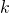with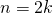." In this version of set-builder notation, the left-hand side (before the pipe) is about what kind of objects the elements of the set being defined are; the right-hand side gives a condition that describes the set.

There is yet another way to use set-builder notation to define a set, as exemplified:

Definition.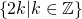We would read this aloud The set of a numbers of the form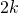, whereis an integer." Notice thathas disappeared entirely from this version. In this version of set-builder notation, the left-hand side gives the general form of the elements of the set, and the right-hand side tells us which elements of that form actually occur in the set.

### Learning to let go of your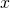s

An open sentence like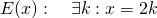has two variables and one quantifier. The two variables are used in quite different ways. First, notice that the only variable that's eligible for plugging in to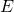is: we can sensibly ask Is it true that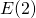? and Is it true that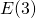?. But substituting forlike this:yields nonsense.

What's going on here? Variables where we can plug in values and get something sensible (likehere) are called free. The other kind of variable (likehere) is called a bound variable, from the verb bind. The idea is that the quantifier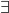has a binding effect on the variable. You can recognize bound variables in three ways:

• Substituting for a bound variable results in nonsense; substituting for a free variable does not.
• Bound variables almost always occur exactly twice; free variables occur once.
• There is always a way to read a mathematical formula containing a bound variable in a way that makes the bound variable go away.

I'll illustrate these checks by giving some examples of bound variables, one of which you've seen before:

Consider the integral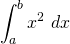The variables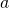and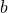are free; the variableis bound.

• We could substitute in forand: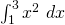. If we substitute for, we get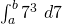, which is nonsense.
• The variableoccurs twice: once in the integrand and once in the differential.
• We could read this integral aloud as The integral, fromto, of the squaring function."

Consider the (true) statement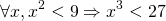Here, the variableis bound and there are no free variables.

• If we tried to substitute, we'd get something like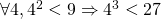, which doesn't make sense.
• The variableoccurs twice: once in the open sentence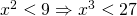, and once in the quantifier.
• We could read this aloud as If a number's square is less than 9, its cube is less than 27."

One very important trick to do with a bound variable is to replace it, across the board, with another variable. I call this letting go of yours. So for example, in an integral we can change the integrating variable at whim: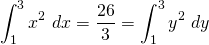We can do the same with quantified statements and open sentences: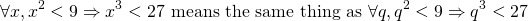What does all this have to do with sets and set-builder notation? Observe that the variables that appear in set-builder notation are almost all bound, which we can tell because they occur twice (once on each side of the pipe). For example: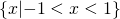is the same set as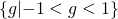which in turn is the same set as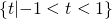The point is that the particular choice of variableor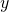or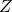or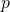or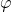has no significance at all. By way of example, if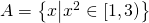, then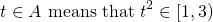In particular, our definition ofdoes not create any particular link between the letterand the set. We could just as well have defined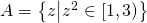.

Published in sets.